# Digits A, B, C

For the various digits A, B, C is true: the square root of the BC is equal to the A and sum B+C is equal to A. Calculate A + 2B + 3C. (BC is a two-digit number, not a product).

Correct result:

A+2B+3C =  28

#### Solution: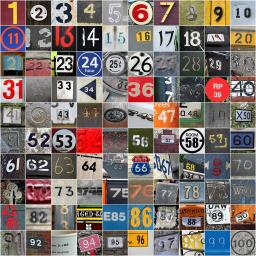We would be pleased if you find an error in the word problem, spelling mistakes, or inaccuracies and send it to us. Thank you!Tips to related online calculators
Do you solve Diofant problems and looking for a calculator of Diofant integer equations?
Do you have a linear equation or system of equations and looking for its solution? Or do you have quadratic equation?

## Next similar math problems:

• Fifth of the numberThe fifth of the number is by 24 less than that number. What is the number?
• PowersExpress the expression ? as the n-th power of the base 10.
• One halfOne half of ? is: ?
• CalculationHow much is sum of square root of six and the square root of 225?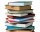Readers borrowed a total of 220 books in the library during the first three days. On the second day, readers borrowed half as many books as the first day and at the same time 20 fewer books than the third day. Depending on the quantity x, express the numb
• Three diceThe player throwing the three dice asked G. Galilei the question: "Should I bet on the sum of 11 or the sum of 12?" What did Galilei answer him? Hint: write down all three triples of numbers that can be thrown and: have a total of 11 have a total of 12 an
• Mom and daughterMother is 39 years old. Her daughter is 15 years. For many years will mother be four times older than the daughter?
• Average ageThe company of five people has an average age of 46 years. The average age of the first four is 43 years. How many years has the fifth member of this company?
• Walnutsx walnuts were in the mission. Dano took 1/4 of nuts Michael took 1/8 from the rest and John took 34 nuts. It stayed here 29 nuts. Determine the original number of nuts.
• Repair company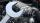The company repairs cars. The first day repair half of the contract second day, the half of the rest and third day 8 residue cars. How many total cars company repaired?
• EquationHow many real roots has equation ? ?
• Bikes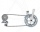Pepa asked Honza how many bikes he had. He replied that he had three-quarters of their total number and another three-quarter of one bike. So how many bikes does Honza have?
• PowerNumber ?. Find the value of x.
• Diofant equation250x + 120y = 5640In six baskets, the seller has fruit. In individual baskets, there are only apples or just pears with the following number of fruits: 5,6,12,14,23 and 29. "If I sell this basket," the salesman thinks, "then I will have just as many apples as a pear." Whic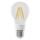One percent of all the lights in the city are LED, the remaining 99% are conventional. Other types are not there. John counted them honestly but he had counted only conventional. After a good dinner he registered numbers and he have notice that from all t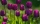Simona picked 63 tulips in the garden and tied bicolor bouquets for her girlfriends. The tulips were only red and white. She put as many tulips in each bouquet, three of which were always red. How much could Simon tear off white tulips? Write all the opti